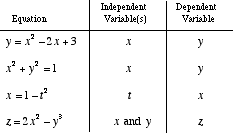index: click on a letter A B C D E F G H I J K L M N O P Q R S T U V W X Y Z A to Z index index: subject areas numbers & symbols sets, logic, proofs geometry algebra trigonometry advanced algebra & pre-calculus calculus advanced topics probability & statistics real world applications multimedia entrieswww.mathwords.com about mathwords website feedback

 Dependent Variable A variable that depends on one or more other variables. For equations such as y = 3x – 2, the dependent variable is y. The value of y depends on the value chosen for x. Usually the dependent variable is isolated on one side of an equation. Formally, a dependent variable is a variable in an expression, equation, or function that has its value determined by the choice of value(s) of other variable(s).See also Independent variable